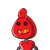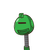# compare the following fractions. write <,>or= in the empty spaces. 5/11_3/8​

compare the following fractions. write <,>or= in the empty spaces. 5/11_3/8​

### 2 thoughts on “compare the following fractions. write <,>or= in the empty spaces. 5/11_3/8​”

1.Step-by-step explanation:

5/11=0.46

3/8=0.375

so the answer is 5/11 >3/8

2.Step-by-step explanation:

5/11 is bigger than 3/8 because when we compare them we need to multiply 5 from 8 and 11 from 3 so we will get 40, 33. Hence, 40 is bigger than 33